• 基于距离相关系数和支持向量机回归的PM_(2.5)浓度滚动统计预报方案[J]. 环境科学学报, 2017,37(4):1268-1276.（我是从这篇论文上找的，维基百科上有更细致的，可惜我看不下去啊） 下为python程序： 原文：...

最近在做特征选择，要考量几个特征的相关性，想找这个方法的描述，发现很难在网页上搜到。以下为整合的：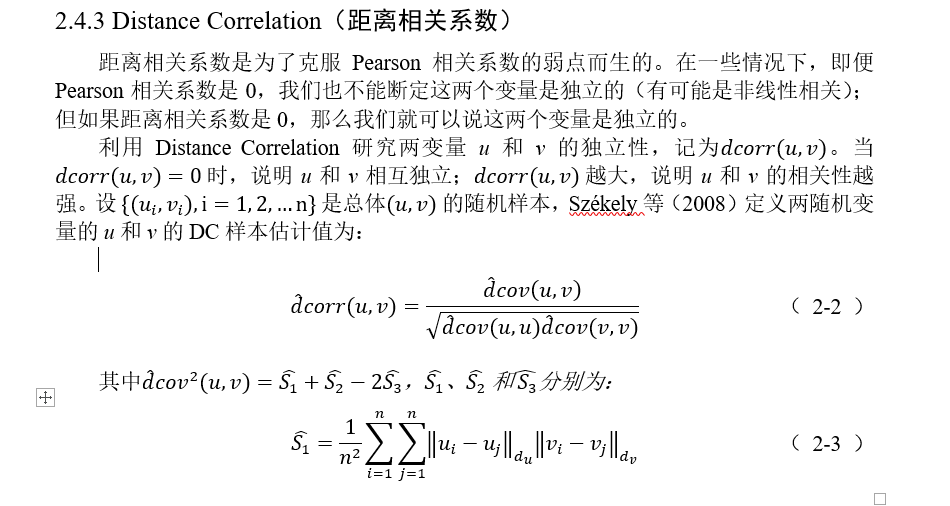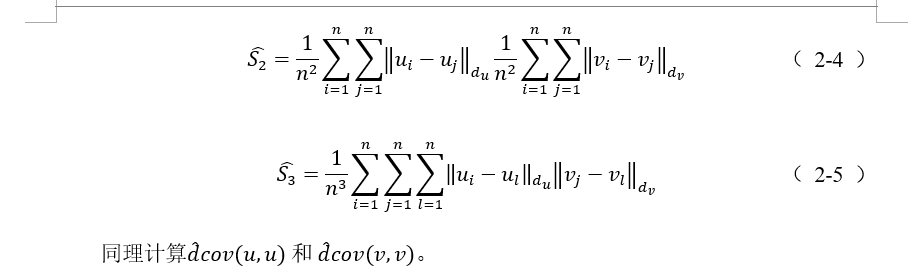王黎明, 吴香华, 赵天良,. 基于距离相关系数和支持向量机回归的PM_(2.5)浓度滚动统计预报方案[J]. 环境科学学报, 2017,37(4):1268-1276.（我是从这篇论文上找的，维基百科上有更细致的，可惜我看不下去啊）

下为python程序：

原文：https://gist.github.com/satra/aa3d19a12b74e9ab7941

from scipy.spatial.distance import pdist, squareform
import numpy as np

from numbapro import jit, float32

def distcorr(X, Y):
""" Compute the distance correlation function

>>> a = [1,2,3,4,5]
>>> b = np.array([1,2,9,4,4])
>>> distcorr(a, b)
0.762676242417
"""
X = np.atleast_1d(X)
Y = np.atleast_1d(Y)
if np.prod(X.shape) == len(X):
X = X[:, None]
if np.prod(Y.shape) == len(Y):
Y = Y[:, None]
X = np.atleast_2d(X)
Y = np.atleast_2d(Y)
n = X.shape
if Y.shape != X.shape:
raise ValueError('Number of samples must match')
a = squareform(pdist(X))
b = squareform(pdist(Y))
A = a - a.mean(axis=0)[None, :] - a.mean(axis=1)[:, None] + a.mean()
B = b - b.mean(axis=0)[None, :] - b.mean(axis=1)[:, None] + b.mean()

dcov2_xy = (A * B).sum()/float(n * n)
dcov2_xx = (A * A).sum()/float(n * n)
dcov2_yy = (B * B).sum()/float(n * n)
dcor = np.sqrt(dcov2_xy)/np.sqrt(np.sqrt(dcov2_xx) * np.sqrt(dcov2_yy))
return dcor

展开全文• 距离相关系数以及python包的安装 觉得有用的话,欢迎一起讨论相互学习~ 版权声明：本文为CSDN博主「 LUC 」的原创文章，遵循CC 4.0 BY-SA版权协议，转载请附上原文出处链接及本声明。 原文链接：...

距离相关系数以及python包的安装

觉得有用的话,欢迎一起讨论相互学习~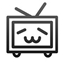版权声明：本文为CSDN博主「 LUC 」的原创文章，遵循CC 4.0 BY-SA版权协议，转载请附上原文出处链接及本声明。
原文链接：https://blog.csdn.net/weixin_45456209/article/details/108356586

距离相关系数：研究两个变量之间的独立性，距离相关系数为0表示两个变量是独立的。克服了皮尔逊相关系数（Pearson）的弱点。pearson相关系数为0并不一定表示两个变量之间是独立的，也有可能是非线性相关的。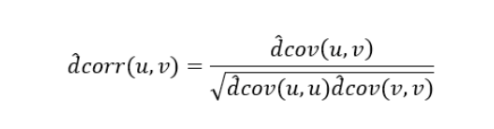python实现：

1.安装dcor包

pip install dcor

安装的时候可能会遇到 报错：ERROR: Cannot uninstall ‘llvmlite’. It is a distutils installed project.

2.使用方法：

import numpy as np
a1=np.array([11,2,56,34])
b1=np.array([45,15,26,24])
dcor.distance_correlation(a1,b1)

out: 0.6673874262718296

这里计算的变量得是array形式，需要使用shape。如果是list则可以转换成array形式进行计算：

import numpy as np
a=[11,2,56,34]
b=[45,15,26,24]
a1=np.array(a)
b1=np.array(b)
dcor.distance_correlation(a1,b1)

References

BCH19
Arin Chaudhuri and Wenhao Hu. A fast algorithm for computing distance correlation. Computational Statistics & Data Analysis, 135:15–24, July 2019. doi:10.1016/j.csda.2019.01.016.

BHS16
Xiaoming Huo and Gábor J. Székely. Fast computing for distance covariance. Technometrics, 58(4):435–447, 2016. URL: http://dx.doi.org/10.1080/00401706.2015.1054435, arXiv:http://dx.doi.org/10.1080/00401706.2015.1054435, doi:10.1080/00401706.2015.1054435.

BSRB07
Gábor J. Székely, Maria L. Rizzo, and Nail K. Bakirov. Measuring and testing dependence by correlation of distances. The Annals of Statistics, 35(6):2769–2794, 12 2007. URL: http://dx.doi.org/10.1214/009053607000000505, doi:10.1214/009053607000000505.

在网上有一个自定义距离相关系数函数的代码：https://gist.github.com/satra/aa3d19a12b74e9ab7941

其中numbapro没有这个包，把numbapro改为numba就好了。

from numba import jit, float32

如果有其他需要补充的和写得不足的地方欢迎各位补充！

展开全文python
• 距离相关系数用来判断两个变量是否独立，值域为[0,2] 值接近0，两个变量正相关 值接近1，两个变量无关 值接近2，两个变量负相关 距离相关系数可以参考：...

距离相关系数用来判断两个变量是否独立，值域为[0,2]

• 值接近0，两个变量正相关
• 值接近1，两个变量无关
• 值接近2，两个变量负相关

维基百科解释：https://en.wikipedia.org/wiki/Distance_correlation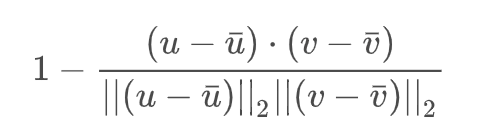python中的使用也很简单：

from scipy.spatial.distance import correlation

if __name__ == '__main__':
corr_values = correlation(
[1, 2, 3, 4, 5],
[5, 4, 3, 2, 5],
)
print(corr_values)

输出1.242535625036333，说明两个序列负相关

特征筛选的示例代码

import pandas as pd
from sklearn.datasets import make_classification

def distance_corr(x_data: pd.DataFrame, y_data: pd.Series) -> pd.DataFrame:
# 距离相关系数
from scipy.spatial.distance import correlation
dis_series = pd.Series(0.0, index=x_data.columns)
for col_name, values in x_data.iteritems():
dis_series[col_name] = correlation(values, y_data)
return pd.DataFrame(dis_series)

if __name__ == '__main__':
value_x, value_y = make_classification(n_samples=1000, n_classes=4, n_features=10, n_informative=8)
df_x = pd.DataFrame(value_x, columns=['f_1', 'f_2', 'f_3', 'f_4', 'f_5', 'f_6', "f_7", "f_8", "f_9", "f_10"])
df_y = pd.Series(value_y)
# 下面是筛选单变量特征
feature_df = distance_corr(df_x, value_y)  # 距离相关系数
for col_index, value in feature_df.iterrows():
print(col_index, ":", value)

展开全文特征工程
• 机器学习：文本相似度计算方法【欧氏距离、余弦距离、皮尔逊相关系数、杰卡德相似系数、KL散度】

相似度就是比较两个事物的相似性。一般通过计算事物的特征之间的距离，如果距离小，那么相似度大；如果距离大，那么相似度小。

问题定义：有两个对象 X X , Y Y ,都包含N维特征， X = ( x 1 , x 2 , x 3 , . . . , x n ) X=(x1,x2,x3,...,xn) , Y = ( y 1 , y 2 , y 3 , . . . , y n ) Y=(y1,y2,y3,...,yn) ,计算X和Y的相似性。

二、曼哈顿距离(Manhattan Distance)

计程车几何 (Taxicab geometry) 或曼哈顿距离 (Manhattan distance or Manhattan length) 或方格线距离是由十九世纪的赫尔曼 · 闵可夫斯基所创辞汇，为欧几里得几何度量空间的几何学之用语，用以标明两个点上在标准坐标系上的绝对轴距之总和

设平面空间内存在两点，它们的坐标为 ( x 1 , y 1 ) ， ( x 2 , y 2 ) (x_1,y_1)，(x_2,y_2)

d i s = ∣ x 1 − x 2 ∣ + ∣ y 1 − y 2 ∣ dis=|x_1-x_2|+|y_1-y_2|

即两点横纵坐标差之和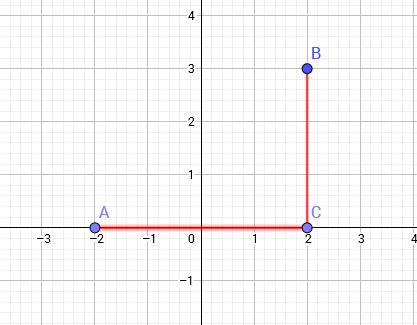如图所示，图中A,B两点的曼哈顿距离为AC+BC=4+3=7

曼哈顿是一个极为繁华的街区，高楼林立，街道纵横，从A地点到达B地点没有直线路径，必须绕道，而且至少要经C地点，走AC和 CB才能到达，由于街道很规则，ACB就像一个直角3角形，AB是斜边，AC和CB是直角边，根据毕达格拉斯(勾股)定理，或者向量理论，都可以知道用AC和CB 可以表达AB的长度。

在早期的计算机图形学中，屏幕是由像素构成，是整数，点的坐标也一般是整数，原因是浮点运算很昂贵，很慢而且有误差，如果直接使用AB的距离，则必须要进 行浮点运算，如果使用AC和CB，则只要计算加减法即可，这就大大提高了运算速度，而且不管累计运算多少次，都不会有误差。因此，计算机图形学就借用曼哈 顿来命名这一表示方法。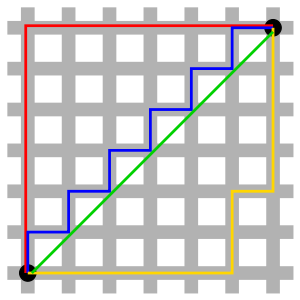曼哈顿与欧几里得距离： 红、蓝与黄线分别表示所有曼哈顿距离都拥有一样长度（12），而绿线表示欧几里得距离有 6 × √ 2 ≈ 8.48 6×√2 ≈ 8.48 的长度。

三、欧氏距离(Euclidean Distance)

两向量 A = [ a 1 , ⋯   , a n ] \mathbf{A}=[{{a}_{1}},\cdots ,{{a}_{n}}] B = [ b 1 , ⋯   , b n ] \mathbf{B}=[{{b}_{1}},\cdots ,{{b}_{n}}] ，这两个向量之间的欧式距离为：

E u c _ d i s t = ∥ A − B ∥ 2 = ∑ i = 1 n ( a i − b i ) 2 = ∑ i = 1 n ( a i 2 − 2 ⋅ a i ⋅ b i + b i 2 ) = ∑ i = 1 n a i 2 + ∑ i = 1 n b i 2 − 2 ⋅ ∑ i = 1 n a i ⋅ b i Euc\_dist={{\left\| \mathbf{A}-\mathbf{B} \right\|}_{2}}=\sqrt{\sum\limits_{i=1}^{n}{{{({{a}_{i}}-{{b}_{i}})}^{2}}}}=\sqrt{\sum\limits_{i=1}^{n}{(a_{i}^{2}-2\centerdot {{a}_{i}}\centerdot {{b}_{i}}+b_{i}^{2})}}=\sqrt{\sum\limits_{i=1}^{n}{a_{i}^{2}}+\sum\limits_{i=1}^{n}{b_{i}^{2}}-2\centerdot \sum\limits_{i=1}^{n}{{{a}_{i}}\centerdot {{b}_{i}}}}

若这两个向量均已归一化，即 ∥ A ∥ 2 = ∑ i = 1 n a i 2 = 1 {{\left\| \mathbf{A} \right\|}_{2}}=\sqrt{\sum\limits_{i=1}^{n}{a_{i}^{2}}}\text{=}1 ∥ B ∥ 2 = ∑ i = 1 n b i 2 = 1 {{\left\| \mathbf{B} \right\|}_{2}}=\sqrt{\sum\limits_{i=1}^{n}{b_{i}^{2}}}\text{=}1

则：
E u c _ d i s t = 1 + 1 − 2 ⋅ ∑ i = 1 n a i ⋅ b i = 2 ⋅ 1 − ∑ i = 1 n a i ⋅ b i Euc\_dist\text{=}\sqrt{1+1-2\centerdot \sum\limits_{i=1}^{n}{{{a}_{i}}\centerdot {{b}_{i}}}}\text{=}\sqrt{2\centerdot 1-\sum\limits_{i=1}^{n}{{{a}_{i}}\centerdot {{b}_{i}}}}

C o s _ d i s = 1 - ∑ i = 1 n a i ⋅ b i Cos\_dis\text{=}1\text{-}\sum\limits_{i=1}^{n}{{{a}_{i}}\centerdot {{b}_{i}}}

进而：
E u c _ d i s t 2 = 2 ⋅ C o s _ d i s = 2 ⋅ ( 1 − C o s _ s i m ) Euc\_dis{{t}^{2}}\text{=}2\centerdot Cos\_dis\text{=}2\centerdot (1-Cos\_sim)

C o s _ s i m = 1 − 1 2 E u c _ d i s t 2 Cos\_sim=1-\frac{1}{2}Euc\_dis{{t}^{2}}

欧式距离越小（越接近0），两向量越相似。

四、切比雪夫距离(Chebyshev Distance)

设平面空间内存在两点，它们的坐标为 ( x 1 , y 1 ) ， ( x 2 , y 2 ) (x_1,y_1)，(x_2,y_2)

d i s = m a x ( ∣ x 1 − x 2 ∣ , ∣ y 1 − y 2 ∣ ) dis=max(|x_1-x_2|,|y_1-y_2|)

即两点横纵坐标差的最大值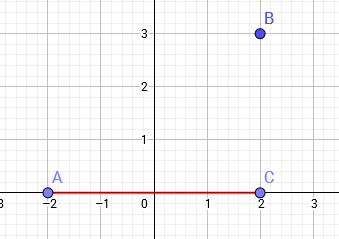d i s = m a x ( A C , B C ) = A C = 4 dis=max(AC,BC)=AC=4

六、余弦相似度(Cosine Similarity)

两向量 A = [ a 1 , ⋯   , a n ] \mathbf{A}=[{{a}_{1}},\cdots ,{{a}_{n}}] B = [ b 1 , ⋯   , b n ] \mathbf{B}=[{{b}_{1}},\cdots ,{{b}_{n}}]

这两个向量之间的余弦相似度Cos_sim为：

C o s _ s i m = A ⋅ B T ∥ A ∥ 2 ⋅ ∥ B ∥ 2 = ∑ i = 1 n a i ⋅ b i ∑ i = 1 n a i 2 ⋅ ∑ i = 1 n b i 2 Cos\_sim\text{=}\frac{\mathbf{A}\centerdot {{\mathbf{B}}^{T}}}{{{\left\| \mathbf{A} \right\|}_{2}}\centerdot {{\left\| \mathbf{B} \right\|}_{2}}}=\frac{\sum\limits_{i=1}^{n}{{{a}_{i}}\centerdot {{b}_{i}}}}{\sqrt{\sum\limits_{i=1}^{n}{a_{i}^{2}}}\centerdot \sqrt{\sum\limits_{i=1}^{n}{b_{i}^{2}}}}

余弦距离为：
C o s _ d i s = 1 − C o s _ s i m = 1 − ∑ i = 1 n a i ⋅ b i ∑ i = 1 n a i 2 ⋅ ∑ i = 1 n b i 2 Cos\_dis\text{=}1-Cos\_sim\text{=}1-\frac{\sum\limits_{i=1}^{n}{{{a}_{i}}\centerdot {{b}_{i}}}}{\sqrt{\sum\limits_{i=1}^{n}{a_{i}^{2}}}\centerdot \sqrt{\sum\limits_{i=1}^{n}{b_{i}^{2}}}}

若这两个向量均已归一化，即 ∥ A ∥ 2 = ∑ i = 1 n a i 2 = 1 {{\left\| \mathbf{A} \right\|}_{2}}=\sqrt{\sum\limits_{i=1}^{n}{a_{i}^{2}}}\text{=}1 ∥ B ∥ 2 = ∑ i = 1 n b i 2 = 1 {{\left\| \mathbf{B} \right\|}_{2}}=\sqrt{\sum\limits_{i=1}^{n}{b_{i}^{2}}}\text{=}1

则：
E u c _ d i s t = 1 + 1 − 2 ⋅ ∑ i = 1 n a i ⋅ b i = 2 ⋅ 1 − ∑ i = 1 n a i ⋅ b i Euc\_dist\text{=}\sqrt{1+1-2\centerdot \sum\limits_{i=1}^{n}{{{a}_{i}}\centerdot {{b}_{i}}}}\text{=}\sqrt{2\centerdot 1-\sum\limits_{i=1}^{n}{{{a}_{i}}\centerdot {{b}_{i}}}}

C o s _ d i s = 1 - ∑ i = 1 n a i ⋅ b i Cos\_dis\text{=}1\text{-}\sum\limits_{i=1}^{n}{{{a}_{i}}\centerdot {{b}_{i}}}

进而：
E u c _ d i s t 2 = 2 ⋅ C o s _ d i s = 2 ⋅ ( 1 − C o s _ s i m ) Euc\_dis{{t}^{2}}\text{=}2\centerdot Cos\_dis\text{=}2\centerdot (1-Cos\_sim)

C o s _ s i m = 1 − 1 2 E u c _ d i s t 2 Cos\_sim=1-\frac{1}{2}Euc\_dis{{t}^{2}}

余弦距离越小（越接近0），两向量越相似。

余弦相似度越大（越接近1），两向量越相似。

十二、Hellinger距离(Hellinger Distance)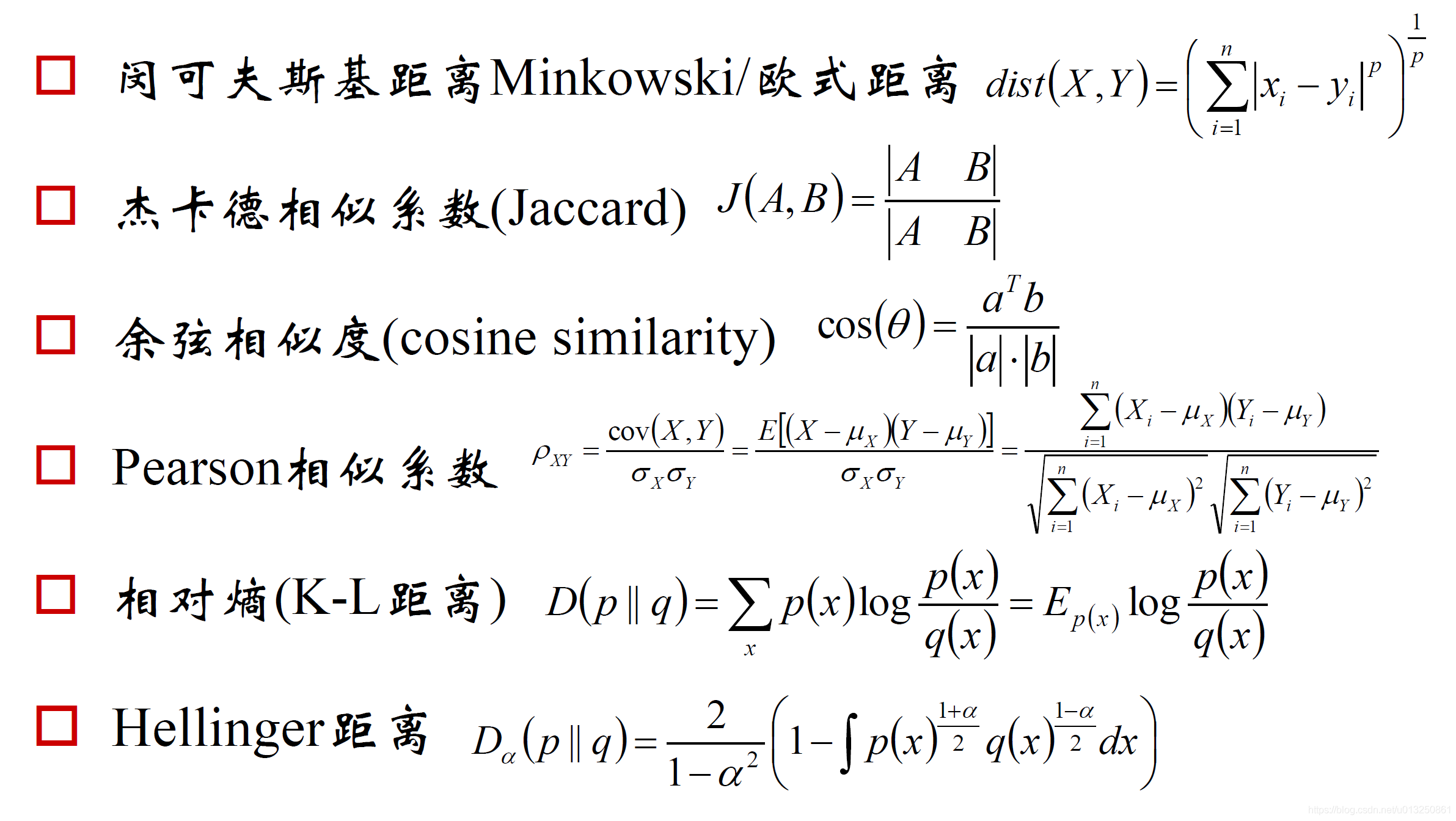展开全文人工智能 自然语言处理 NLP 文本相似度
• 本帖最后由 老姜 于 2013-12-11 03:30 编辑我做了个非线性拟合，结果发现：相关系数之平方(R^2) 不等于决定系数(DC)，自己采用1-SSE/SST计算后得到DC值，想知道lstopt 的R^2是如何计算？原代码如下：Function z=(a*b...
• 还可以计算：D(X)=E(X^2)-E^2(X)=(1.1^2+1.9^2+3^2)/3 - 4=4.60-4=0.6 σx=0.77 D(Y)=E(Y^2)-E^2(Y)=(5^2+10.4^2+14.6^2)/3-100=15.44 σy=3.93 X,Y的相关系数： r(X,Y)=Cov(X,Y)/(σxσy)=3.02/(0.77×3.93) = 0....
• netflix推荐电影的基本逻辑是，如果我对某电影打了高分或者低分，那么他就寻找同样对该视频打高分或者低分的人，...取值范围 [-1, 1].* 1: 完全正相关* 0：不存在相关性* -1：完全负相关相关系数计算的原理：* 如果...
• 相关系数图可视化

千次阅读 2021-04-22 14:21:48
相关系数图junjun2016年4月6日参考：刘顺祥作品虽然cor()函数可以非常方便快捷的计算出连续变量之间的相关系数，但当变量非常多时，返回的相关系数一定时读者看的眼花缭乱。下面就以R自带的mtcars数据集为例，讲讲...
• 最常用的相关系数全称是皮尔逊相关系数，两个变量X和Y之间的相关程度，准确来说是线性相关程度。 。分子是X，Y的协方差，分母是X，Y各自的方差，其值介于-1与1之间。可以观察到相关系数与数据形状和方向之间的...自相关系数
• 前面我们已经讨论了 Pearson 相关系数和 Spearman 秩相关系数，它们可以检测连续变量间的相关性，并且 Spearman 秩相关系数还能够检测有序的离散变量间的相关系数。今天我们再讨论一个能够检测有序变量相关性的系数...
• 目录Pearson相关系数定义Matlab中的相关函数corrcoef和corr计算两个变量Pearson相关系数随时间变化的例子计算两个变量Pearson相关系数随时间变化的例子如何插入一段漂亮的代码片生成一个适合你的列表创建一个表格...matlab 算法
• 相关系数

2021-02-08 23:02:33
excel：CORREL() 括号里面放两列距离，测试是正相关还是负相关相关系数
• 皮尔森相关系数也称皮尔森积矩相关系数(Pearson product-moment correlation coefficient) ，是一种线性相关系数，是最常用的一种相关系数。记为r，用来反映两个变量X和Y的线性相关程度，r值介于-1到1之间，绝对值越...数据评估
• 目录LpL_pLp​ 距离 (LpL_pLp​ distance) / Minkowski 距离 (Minkowski distance)马氏距离 / 马哈拉诺比斯距离 (Mahalanobis Distance) LpL_pLp​ 距离 (LpL_pLp​ distance) / Minkowski 距离 (Minkowski distance...机器学习
• 在统计学中，皮尔逊相关系数( Pearson correlation coefficient），又称皮尔逊积矩相关系数（Pearson product-moment correlation coefficient，简称 PPMCC或PCCs），是用于度量两个变量X和Y之间的相关程度（线性...机器学习 线性代数
• 【判断题】扩展库numpy的函数corrcoef()用来计算相关系数矩阵。 【单选题】下列关于二叉树模型的表述中,错误的是 【判断题】化学家,一般是指从事化学研究的科学家。 【单选题】哪一种计算Var的方法在计量期权风险时...
• 使用相关矩阵对数据进行聚类是一个合理的想法,但必须首先预先处理相关性.首先,numpy.corrcoef返回的相关矩阵受到机器算术错误的影响：>它并不总是对称的.>对角线术语并不总是正好1这些可以通过使用转置取平均...
• Pearson和Spearman相关系数一、皮尔逊(Pearson)相关系数二、Pearson相关系数的Python实例斯皮尔曼(Spearman）相关系数Python实例 一、皮尔逊(Pearson)相关系数 1、协方差能完美的解释两个变量之间相关的方向，但在...
• 皮尔逊相关系数R语言例子理解皮尔逊相关的两个角度其一, 按照高中数学水平来理解, 皮尔逊相关(Pearson Correlation Coefficient)很简单,可以看做将两组数据首先做Z分数处理之后,然后两组数据的乘积和除以样本数Z分数...
• torch.cosine_similarity 可以对两个向量或者张量计算相似度 ...Hamming 距离，汉密尔顿距离 torch.dist(hg1, hg2, p=1) ———————————————— 版权声明：本文为CSDN博主「东方小烈」的原创文...pytorch 人工智能 python
• 这个系数和欧式距离、皮尔逊相关系数等类似，都是度量两个物理量（随机变量）的距离。 1 定义 归一化相关系数度量体现两个向量的夹角。 我们来看一下它的定义式： 2 应用 2.1 衡量图片相关度 NCC是一种基于统计学...机器学习
• 皮尔森相关系数也称皮尔森积矩相关系数(Pearson product-moment correlation coefficient) ，是一种线性相关系数，是最常用的一种相关系数。记为r，用来反映两个变量X和Y的线性相关程度，r值介于-1到1之间，绝对值越...数据分析
• @xhinking皮尔逊相关系数理解有两个角度其一, 按照高中数学水平来理解, 它很简单, 可以看做将两组数据首先做Z分数处理之后, 然后两组数据的乘积和除以样本数Z分数一般代表正态分布中, 数据偏离中心点的距离....
• 这一篇我们来聊聊大家平常比较常用的相关系数。...相关系数主要有三种：Pearson相关系数、Spearman秩相关系数和Kendall τ相关系数。皮尔逊(Pearson)相关系数大家应该都知道，也应该有用到过。但是秩相关(Spearman...
• 1. 概念 pearson相关系数衡量的是线性相关关系。若系数等于0，只能说无线性相关关系，不能说无相关关系。相关系数的绝对值越大，相关性越强：相关系数越接近于1或-1，相关度越强，相关系数越接近于0，相关度越弱。 ...
• 在分析指标与指标、指标与研究对象的影响程度时，很多时候会用到相关系数法，下面介绍一下斯皮尔曼相关系数法。 斯皮尔曼等级相关是根据等级资料研究两个变量间相关关系的方法。它是依据两列成对等级的各对等级数...matlab
• 使用系数

2021-06-24 03:15:14
使用系数是设备的使用时间与允许使用时间的比值。通常以一年累积的时间为计算范围。使用系数x年使用小时基数=年使用小时数。例如，一台电机每天工作八小时，一年50周，那么一年电机工作的时候是2000小时。如果电机是...
• 相关系数简记

2021-02-10 11:15:32
1.连续数据，正态分布，线性关系，用pearson相关系数是最恰当，当然用spearman相关系数也可以，效率没有pearson相关系数高。 2.上述任一条件不满足，就用spearman相关系数，不能用pearson相关系数。 3.两个定序测量......